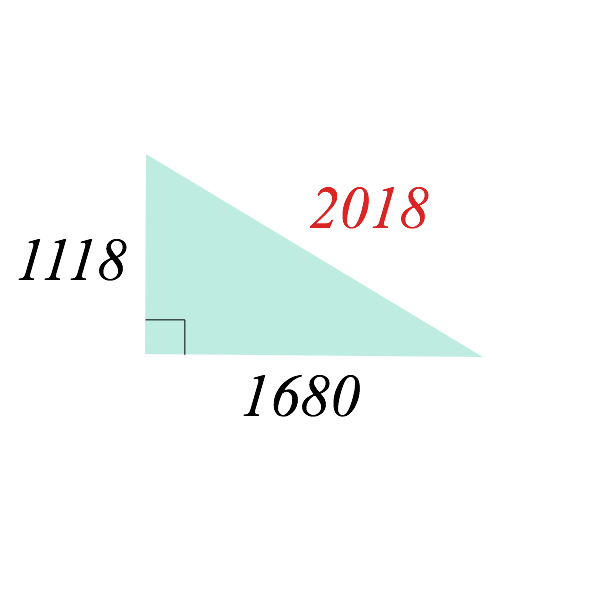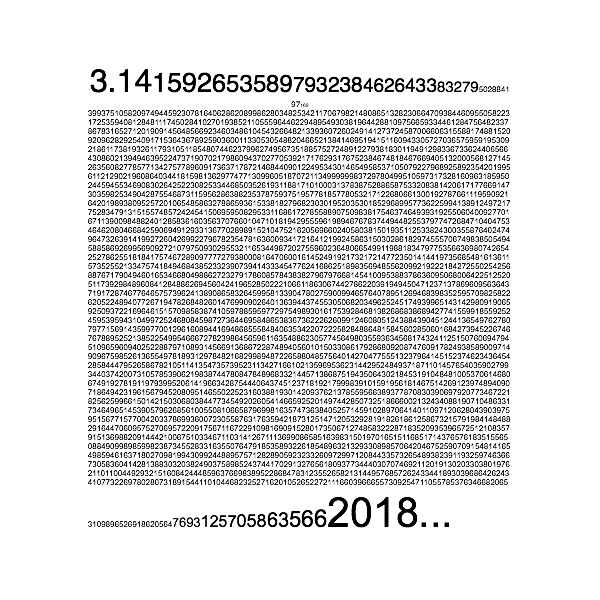# Mathematics About the Number 2018Happy New Year 2018!!

Here are several mathematical facts about the number 2018.Add to solve later

## Is 2018 a Prime Number?

The number 2018 is an even number, so in particular 2018 is not a prime number.
The prime factorization of 2018 is
$2018=2\cdot 1009.$ Here $2$ and $1009$ are prime numbers.

## Identities for 2018

Here are some identities for 2018.

### 2018 is the sum of two squares

2018 is the sum of two squares:
\begin{align*}
2018=13^2+43^2.
\end{align*}
Here are some variants.
\begin{align*}
2018&=1^2+9^2+44^2\\
2018&=1^2+12^2+28^2+33^2\\
2018&=2^2+2^2+5^2+7^2+44^2\\
2018&=6^2+2(29)^2+3(10)^2
\end{align*}

### 2018 is a part of a Pythagorean triple

2018 is a part of a Pythagorean triple:
\begin{align*}
2018^2=1118^2+1680^2.
\end{align*}

This means that there is a right triangle whose hypotenuse is 2018.Here are some variants.
\begin{align*}
2018^2&=18^2+88^2+2016^2\\
2018^2&=460^2+566^2+1172^2+1472^2\\
2018^3&=421^3+1490^3+1691^3
\end{align*}

## 2018 is the sum of 12 successive integers

2018 is the sum of 12 successive integers:
$2018=7^2+8^2+9^2+10^2+11^2+12^2+13^2+14^2+15^2+16^2+17^2+18^2.$

## 2018 appears in $\pi=3.14…$

2018 appears in the number $\pi=3.14…$ as in the following picture.Did you miss mathematical facts about 2017?

Check outAdd to solve later

### 1 Response

1. 01/01/2018

[…] Check out the post Mathematics about the number 2018 […]

This site uses Akismet to reduce spam. Learn how your comment data is processed.

###### More in General##### Top 10 Popular Math Problems in 2016-2017

It's been a year since I started this math blog!! More than 500 problems were posted during a year (July...

Close